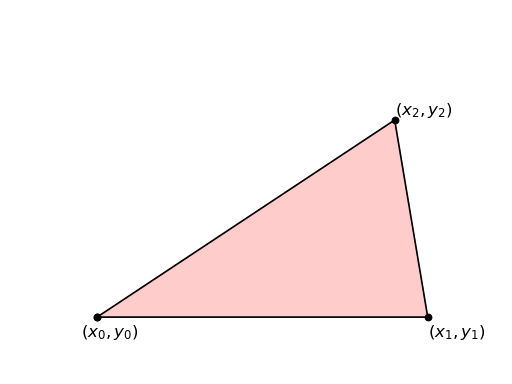Quadrature is a Python module for computing the quadratures (integrals) of homogenous polynomials of arbitrary order over any simplices in any dimension.

Suppose we are given a k-Simplexinwhererepresents the ith vertex of the simplex.We are interested in finding an explicit expression for the quadrature of a homogeneous polynomial over such a simplex:and the moments, which are the above expression divided by the volume of the simplex:We have implemented in python a simple algorithm that computes exactly the above expressions:

`quadrature.``nCk`(n, k)[source]

Compute the binomial coefficients```>>> nCk(0,0)
1
>>> nCk(1,0)
1
>>> nCk(4, 2)
6
>>> [[nCk(n,k) for k in range(n+1)] for n in range(7)] == [
...          ,
...        [1, 1],
...       [1, 2, 1],
...      [1, 3, 3, 1],
...     [1, 4, 6, 4, 1],
...    [1, 5, 10, 10, 5, 1],
...  [1, 6, 15, 20, 15, 6, 1]                              ]
True
```
`quadrature.``quadrature`(term, verts)[source]

Compute the quadrature of a homogenous polynomial given by term=’xxy’ over the vertices verts=(0,1,2) of a simplex.

```>>> quadrature('', (0,1))
([((), 1)], Fraction(1, 1))
([((('x', 0),), 1), ((('x', 1),), 1)], Fraction(1, 2))
([((('x', 0),), 1), ((('x', 1),), 1), ((('x', 2),), 1)], Fraction(1, 3))
...    [((('x', 0), ('x', 0)), 1),
...     ((('x', 0), ('x', 1)), 1),
...     ((('x', 1), ('x', 1)), 1)], fractions.Fraction(1, 3))
True
...    [((('x', 0), ('y', 0)), 2),
...      ((('x', 0), ('y', 1)), 1),
...      ((('x', 0), ('y', 2)), 1),
...      ((('x', 1), ('y', 0)), 1),
...      ((('x', 1), ('y', 1)), 2),
...      ((('x', 1), ('y', 2)), 1),
...      ((('x', 2), ('y', 0)), 1),
...      ((('x', 2), ('y', 1)), 1),
...      ((('x', 2), ('y', 2)), 2)], fractions.Fraction(1, 12))
True
```

## Description of Algorithm¶

Our algorithm is general, but for simplicity we will just demonstrate it for the specific case of a triangle in two dimensions. Assume you have a triangle in the plane defined by its three vertices at,, and- it is a 2-simplex so. We will compute the moment of the q-homoqenous polynomialwith.The inputs to the algorithm are two tuples - the first isdescribing the polynomial and the other isdescribing the labels for the vertices. With this notation, the orders of the simplexand the degree of the polynomialare given byNow take all the permutations of thetuple.Compute all the combinations (with repetitions allowed) of sizeof the coordinates of the verticesTake the product ofandFor each element in the tuple zip together the variable with the simplex vertices (e.g.and so on):Treat each element as a multiplication and sum all the multiples togetherDivide the result byto obtain the final expressionwhich is indeed what one would obtain forby manual calculation.

## Pseudocode¶

In pseudocode the algorithm can be concisely given as:## Validation¶

To validate the algorithm we consider a triangle, a line segment, and a tetrahedron, and we compute a number of low order moments by integrating explicitly. The Mathematica file where all the integration is done is provided `for download here`.

### Trianglein¶

The moments of all the homogeneous polynomials over a triangle area given bywhereis the area form forandis the total area of the triangle.

For example,andare the coordinates of the center of mass of the triangle.

Change of variablesso thatcorresponds to vertex,corresponds to vertex, andcorresponds to vertex. The area form transforms as follows:Thus we have an explicit expression for the quadraturewhich can be evaluated by hand to obtain the first few moments:Those match exactly with the results from our algorithm.

`quadrature.``test_triangle`()[source]
```>>> def q(t): return tostr(quadrature(t, (0,1,2)))
>>> q('x')
'1/3 (x0 + x1 + x2)'
>>> q('xx')
'1/6 (x0x0 + x0x1 + x0x2 + x1x1 + x1x2 + x2x2)'
>>> q('xxx')
'1/10 (x0x0x0 + x0x0x1 + x0x0x2 + x0x1x1 + x0x1x2 + x0x2x2 + x1x1x1 + x1x1x2 + x1x2x2 + x2x2x2)'
>>> q('xy')
'1/12 (2 x0y0 + x0y1 + x0y2 + x1y0 + 2 x1y1 + x1y2 + x2y0 + x2y1 + 2 x2y2)'
```

### Line Segmentin¶

The same be done for line segments defined by their endpointsand. Again the first few moments in this case are:And our algorithm gives

`quadrature.``test_line_segment`()[source]
```>>> def q(t): return tostr(quadrature(t, (0,1)))
>>> q('x')
'1/2 (x0 + x1)'
>>> q('xx')
'1/3 (x0x0 + x0x1 + x1x1)'
>>> q('xxx')
'1/4 (x0x0x0 + x0x0x1 + x0x1x1 + x1x1x1)'
>>> q('xy')
'1/6 (2 x0y0 + x0y1 + x1y0 + 2 x1y1)'
>>> q('xxy')
'1/12 (3 x0x0y0 + x0x0y1 + 2 x0x1y0 + 2 x0x1y1 + x1x1y0 + 3 x1x1y1)'
```

### Tetrahedronin¶`quadrature.``test_tetrahedron`()[source]
```>>> def q(t): return tostr(quadrature(t, (0,1,2,3)))
>>> q('x')
'1/4 (x0 + x1 + x2 + x3)'
>>> q('xx')
'1/10 (x0x0 + x0x1 + x0x2 + x0x3 + x1x1 + x1x2 + x1x3 + x2x2 + x2x3 + x3x3)'
>>> q('xxx')
'1/20 (x0x0x0 + x0x0x1 + x0x0x2 + x0x0x3 + x0x1x1 + x0x1x2 + x0x1x3 + x0x2x2 + x0x2x3 + x0x3x3 + x1x1x1 + x1x1x2 + x1x1x3 + x1x2x2 + x1x2x3 + x1x3x3 + x2x2x2 + x2x2x3 + x2x3x3 + x3x3x3)'
>>> q('xy')
'1/20 (2 x0y0 + x0y1 + x0y2 + x0y3 + x1y0 + 2 x1y1 + x1y2 + x1y3 + x2y0 + x2y1 + 2 x2y2 + x2y3 + x3y0 + x3y1 + x3y2 + 2 x3y3)'
```